# SAT Math: Equations and Expressions Chapter Exam

Exam Instructions:

Choose your answers to the questions and click 'Next' to see the next set of questions. You can skip questions if you would like and come back to them later with the yellow "Go To First Skipped Question" button. When you have completed the practice exam, a green submit button will appear. Click it to see your results. Good luck!

### Page 1

#### Question 5 5. What is the b-value of the quadratic equation below?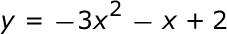### Page 2

#### Question 7 7. Which of the following is a solution to the system of equations shown in the graph below?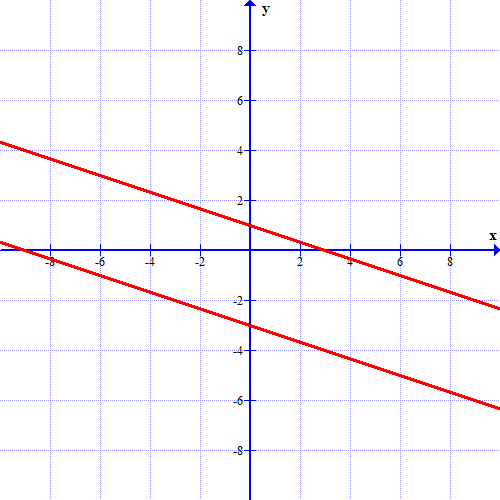### Page 3

#### Question 13 13. Find the roots of the equation.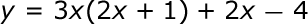#### Question 14 14. Rewrite the quadratic below in standard form: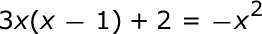### Page 6

#### Question 26 26. What would the first step be in completing the square of the following equation?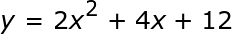#### Question 27 27. Find the solution to the equation below.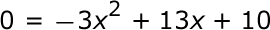#### Question 29 29. Simplify: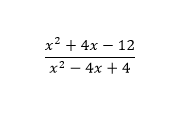#### SAT Math: Equations and Expressions Chapter Exam Instructions

Choose your answers to the questions and click 'Next' to see the next set of questions. You can skip questions if you would like and come back to them later with the yellow "Go To First Skipped Question" button. When you have completed the practice exam, a green submit button will appear. Click it to see your results. Good luck!

Support